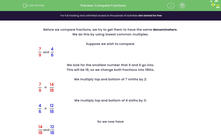# Compare Fractions by Finding the Common Denominator

In this worksheet, students will use common denominators to compare fractions.Key stage:  KS 2

Curriculum topic:   Number: Fractions, Decimals and Percentages

Curriculum subtopic:   Compare and Order Fractions

Popular topics:   Fractions worksheets

Difficulty level:#### Worksheet Overview

Before we compare fractions, we try to get them to have the same denominators.

We do this by using lowest common multiples.

Suppose we wish to compare:

 7 and 4 9 6

We look for the smallest number that 9 and 6 go into.

This will be 18, so we change both fractions into 18ths.

We multiply the numerator and denominator of 7/9 by 2:

 7 = 14 9 18

We multiply the numerator and the denominator of 4/6 by 3:

 4 = 12 6 18

So we now have

 14 and 12 18 18

 14 > 12 18 18

 7 > 4 9 6

Example

Which is bigger?

 12 or 11 8 6

The lowest common multiple of 8 and 6 is 24, because 3 × 8 = 24 and 4 × 6 = 24.

We multiply the numerator and the denominator of 12/8 by 3:

 12 = 36 8 24

We multiply the numerator and the denominator of 11/6 by 4:

 11 = 44 6 24

 44 > 36 24 24

 11 is bigger 6

Let's move on to the questions now.

### What is EdPlace?

We're your National Curriculum aligned online education content provider helping each child succeed in English, maths and science from year 1 to GCSE. With an EdPlace account you’ll be able to track and measure progress, helping each child achieve their best. We build confidence and attainment by personalising each child’s learning at a level that suits them.

Get started